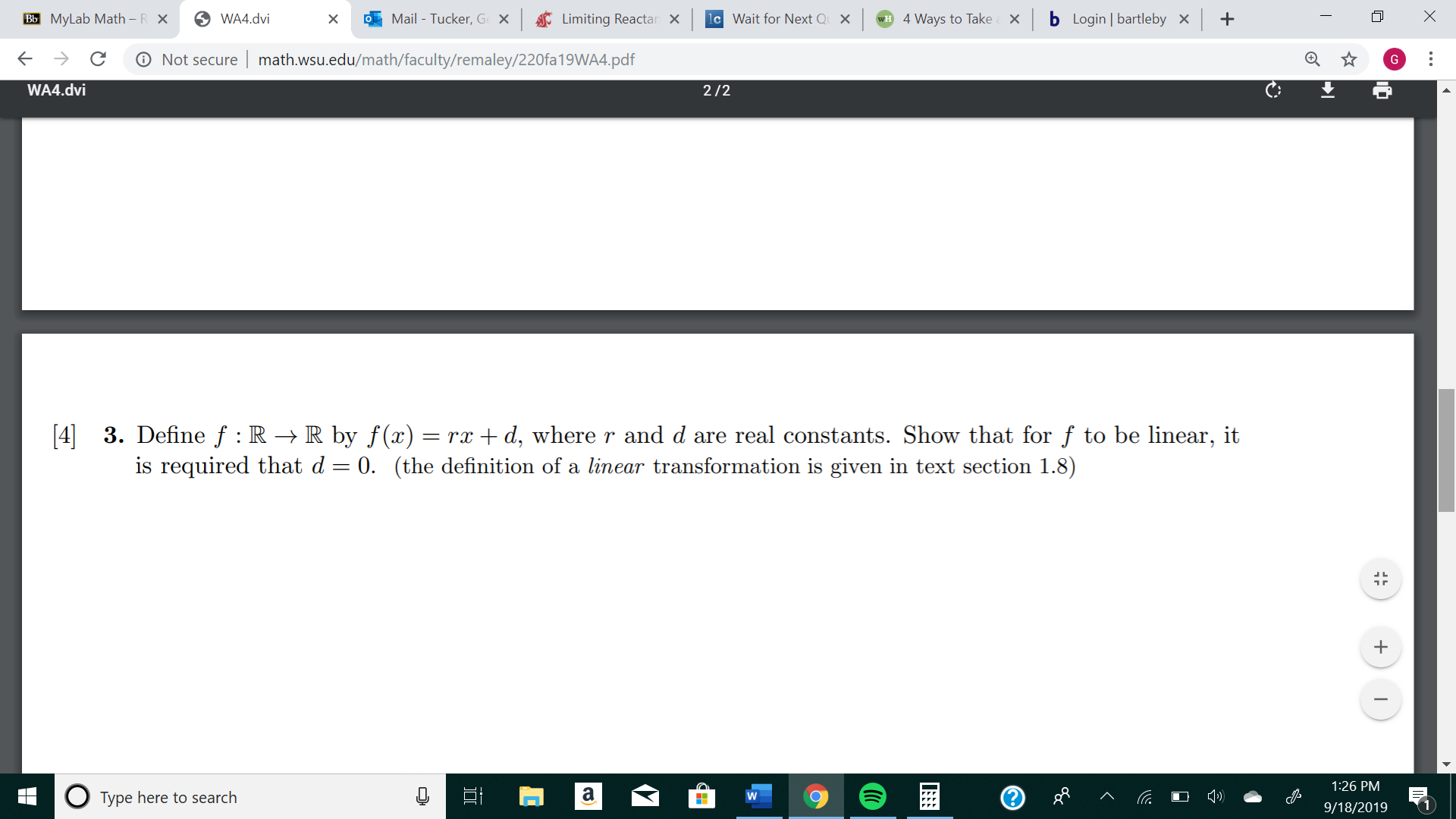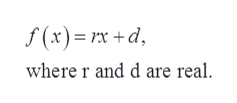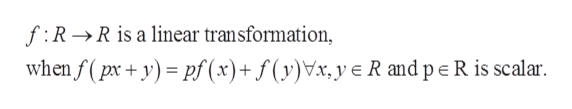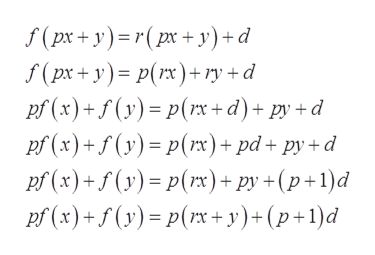Mail Tucker, Ge xLimiting Reactanlc Wait for Next Q Xb Login | bartlebyBb MyLab Math - R XWA4.dviwH 4 Ways to TakeO Not securemath.wsu.edu/math/faculty/remaley/220fa19WA4.pdfGWA4.dvi2/2 3. Define f R- R by f(x)= rx d, where r and d are real constants. Show that for f to be linear, itis required that d 0. (the definition of a linear transformation is given in text section 1.8)+1:26 PMaType here to search9/18/2019X...

Questionhelp_outlineImage TranscriptioncloseMail Tucker, Ge x Limiting Reactan lc Wait for Next Q X b Login | bartleby Bb MyLab Math - R X WA4.dvi wH 4 Ways to Take O Not secure math.wsu.edu/math/faculty/remaley/220fa19WA4.pdf G WA4.dvi 2/2  3. Define f R- R by f(x) = rx d, where r and d are real constants. Show that for f to be linear, it is required that d 0. (the definition of a linear transformation is given in text section 1.8) + 1:26 PM a Type here to search 9/18/2019 X ... fullscreen
Step 1

Consider the linear function.help_outlineImage Transcriptionclosef(x) x +d where r and d are real fullscreen
Step 2

Since, a functionhelp_outlineImage Transcriptionclosef RR is a linear transformation, when f (px+ypf (x)+f(y)Vx,ye R and pe R is scalar fullscreen
Step 3

Therefore...help_outlineImage Transcriptionclosef(px+ y)r(px+y)+ d f(px+y) p(rx)ry d pf (x)+f(y)p(+d)+pyd pf (x)+f(y)p()+ pd+ py+d pf (x)+f(y) p(x)+ py +(p+1)d pf (x)+y) p(rx+ y)+(p+1)d fullscreen

Want to see the full answer?

See Solution

Want to see this answer and more?

Our solutions are written by experts, many with advanced degrees, and available 24/7

See Solution
Tagged in

Algebra